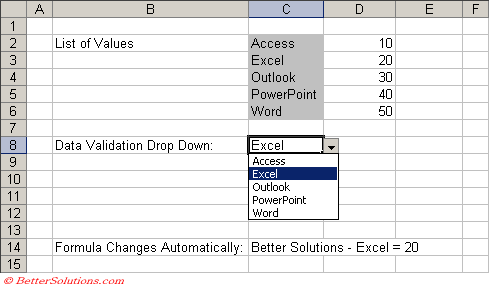# VBA Code

`Selection.Validation.Add(Type:=xlDVType.xlValidateList                          AlertStyle:=xlDVAlertStyle.xlValidAlertStop, _                          Operator:=xlFormatConditionOperator.xlBetween, _                          Formula1:="A,B,C,D", _                          Formula2:= `

`With Selection.Validation     .Delete     .IgnoreBlank = True     .InCellDropdown = True     .ShowInput = True End With `

This can even be abbreviated to the following:

`Selection.Validation.Add Type:=xlValidateList, Formula1:="Excel,Word,PowerPoint" `

### Responding to a Data Validation Drop Down

It is possible to run a macro when the value is changed from a Data Validation drop-down box.
You can use the Worksheet_Change() event to detect when the value in the drop-down box has changed.

`Private Sub Worksheet_Change(ByVal Target As Range) Dim irowno As Integer    If Target.Column = 3 And _       Target.Row = 8 Then             irowno = Application.WorksheetFunction.Match(Range("C8").Value, Range("C2:C6"))       Range("C14").Formula = "Better Solutions - " & Range("C8").Value & " = " & Range("D" & 1 + irowno).Value    End If End Sub `© 2023 Better Solutions Limited Prev